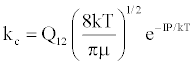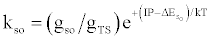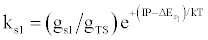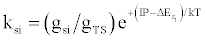Reaction Rate constant* (a) M+(KE=kTg) +e*(KE=IP) → (M+-e*)(KE=IP) ↔ (M+e-)*(KE=IP)(b) (M+e-)* → M+hn(so)(c) (M+e-)* → M+hn(s1)(d) (M+e-)* → M+hn(si)*kso and ks1 are the rate constants for radiative relaxation to the so and s1 electronic states, IP=ionization potential of M, and ΔEso, ΔEs1 and ΔEsi are the energy differences between the so, s1 and si electronic states with the ground state, so, and gsi and gTS are the statistical weights of the Si state and ambipolar diffusion ionelectron transition state, respectively (Source: See Ref. )
Table 5: Rate constants for Steps involved in collisional radiative recombination.
Goto home»